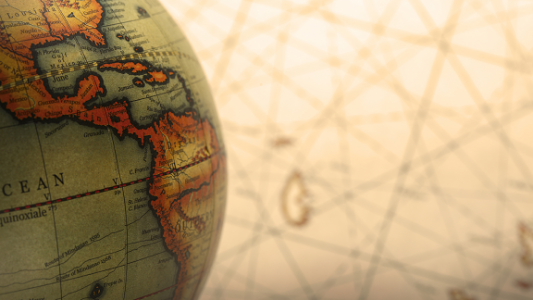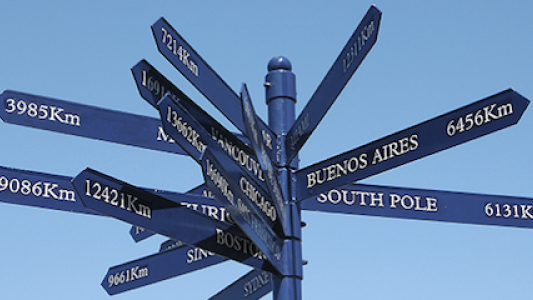# Infinity

Infinity is literally one of the biggest ideas in mathematics! We invite you to explore these problems that lead you to consider the awe and wonder of the infinite.### Where Can We Visit?

Put a counter on a 100-square, and then move it around using only two operations. Which numbers can you visit?### Are We Nearly There?

Try to visualise the route taken by the arrows in our animation. What patterns do you notice?### Odds, Evens and More Evens

Alison, Bernard and Charlie have been exploring sequences of odd and even numbers, which raise some intriguing questions...### What is infinity?

What do we mean by infinity? Did you know there's more than one kind of infinity? Read this article and all will be revealed!### Countable infinities

An infinite set is called countable if you can count it. Read this article to find out how to count infinite sets!### Counting numbers

Are there more irrational numbers than rational numbers, or more rational numbers than irrational numbers? How can we make sense of questions like this about infinity?